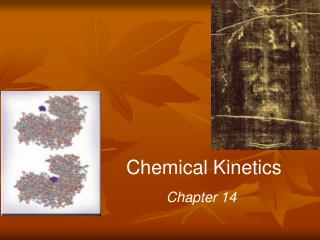# Chemical Kinetics - PowerPoint PPT PresentationDownload PresentationChemical Kinetics

Chemical KineticsDownload Presentation## Chemical Kinetics

- - - - - - - - - - - - - - - - - - - - - - - - - - - E N D - - - - - - - - - - - - - - - - - - - - - - - - - - -
##### Presentation Transcript

1. Chemical Kinetics Chapter 14

2. Chemical Kinetics Thermodynamics – does a reaction take place? Kinetics – how fast does a reaction proceed?

3. Factors That Affect Reaction Rates • Physical State of the Reactants • Concentration of Reactants • Temperature • Presence of a Catalyst Fig 14.2

4. A B rate = D[A] D[B] rate = − Dt Dt Reaction rate - the change in the concentration of a reactant or a product with time (M/s) D[A] = change in concentration of A over time period Dt Because [A] decreases with time, D[A] is negative D[B] = change in concentration of B over time period Dt

5. rate = D[A] D[B] rate = − Dt Dt Fig 14.3 Progress of a hypothetical reaction A→B

6. Change of Rate with Time C4H9Cl (aq) + H2O (l)→ C4H9OH (aq) + HCl (aq) • Average rate decreases as reaction proceeds • As the reaction goes forward, there are fewer collisions between reactant molecules

7. d[CH4] rate = − dt Fig 14.4 Concentration of butylchloride as a function of time Initial rate ≡ rate at t = 0 • Instantaneous rate ≡ slope of line tangent to the curve at any point

8. −[C4H9Cl] t Rate = = [C4H9OH] t Reaction Rates and Stoichiometry C4H9Cl (aq) + H2O (l)→ C4H9OH (aq) + HCl (aq) • In this reaction, the ratio of C4H9Cl to C4H9OH is 1:1 • Rate of consumption of C4H9Cl = rate of formation of C4H9OH

9. 1 2 [HI] t Rate = − = [I2] t Reaction Rates and Stoichiometry • What if the ratio is not 1:1? 2 HI (g) → H2 (g) • In such a case:

10. aA + bB cC + dD = = rate = − = − Δ[C] Δ[B] Δ[D] Δ[A] 1 1 1 1 Δt Δt Δt Δt c b a d Reaction Rates and Stoichiometry Eqn [14.4]

11. Br2(aq) + HCOOH (aq) 2Br −(aq) + 2H+(aq) + CO2(g) time Fig 14.5 Basic components of a spectrophotometer Br2(aq) Br −(aq)

12. Br2(aq) + HCOOH (aq) 2Br −(aq) + 2H+(aq) + CO2(g) time Br2(aq) 393 nm Br2(aq) Br −(aq) Beer’s Law: A = abc D[Br2] D Absorption

13. aA + bB cC + dD The Rate Law Rate law - expresses the relationship of the rate of a reaction to the rate constant and the concentrations of the reactantsraised to some powers Rate = k [A]x[B]y reaction is xth order in A reaction is yth order in B reaction is (x +y)th order overall

14. F2(g) + 2ClO2(g) 2FClO2(g) 1 Rate Laws • Rate laws always determined experimentally • Reaction order always defined in terms of reactant (not product) concentrations • Order of a reactant is not related to the stoichiometric coefficient of the reactant in the balanced chemical equation rate = k [F2][ClO2]

15. Br2(aq) + HCOOH (aq) 2Br −(aq) + 2H+(aq) + CO2(g) rate k = [Br2] Plot of rate vs [Br2] rate  [Br2] rate = k [Br2] y = mx + b = rate constant = 3.50 x 10-3 s-1